# Angateeah

•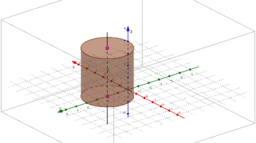### To display cylinder with given axis and radius

Activity

Angateeah

•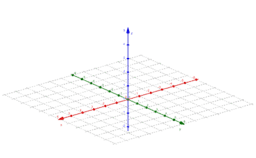### Foot of perpendicular and perpendicular distance of a point from a line

Activity

Angateeah

•### Dot product and cross product

Activity

Angateeah

•### Point of intersection and angle between 2 lines

Activity

Angateeah

•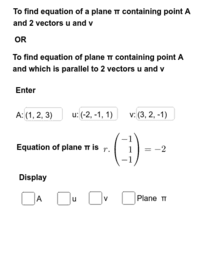### Equation of plane given a point on the plane and 2 vectors

Activity

Angateeah

•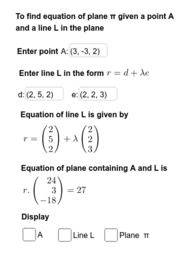### Equation of plane given a point and a line in the plane

Activity

Angateeah

•### Equation of plane given a line in the plane and a perpendicular plane

Activity

Angateeah

•### Equation of plane given a line in the plane and a parallel line

Activity

Angateeah

•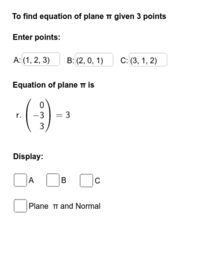### Equation of plane given 3 points

Activity

Angateeah

•### Equation of line in the form r = a + t b

Activity

Angateeah

•### Equation of line given 2 points or a point and a vector

Activity

Angateeah

•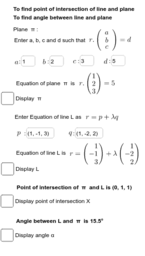### To find the point of intersection and angle between line and plane

Activity

Angateeah

•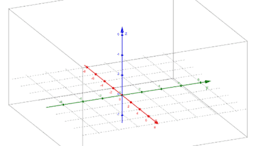### Mid-point and Segment formula

Activity

Angateeah

•### Mid-point, Modulus, Unit Vector

Activity

Angateeah

•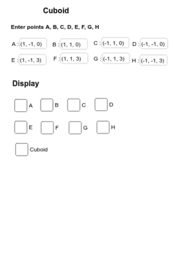### Cuboid

Activity

Angateeah

•### Pyramid

Activity

Angateeah

•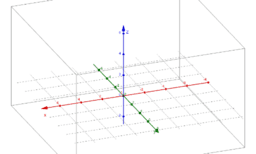### Equation of line, parallel and perpendicular lines

Activity

Angateeah

•### 3 D Vector

Book

Angateeah

•### Equation of line of Intersection between 2 planes

Activity

Angateeah

•### To verify whether 3 points are collinear

Activity

Angateeah

•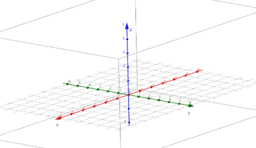### Dot product and angle between 2 vectors

Activity

Angateeah

•### Mid-point, Modulus, Unit Vector

Activity

Angateeah

•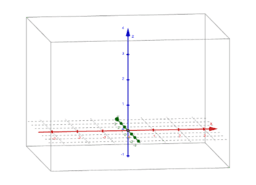### Pyramid

Activity

Angateeah

•Activity

Angateeah